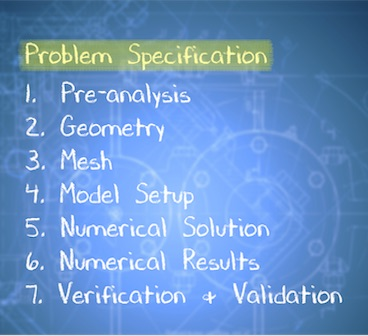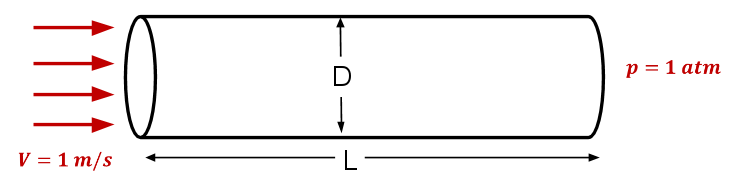# Page History

## Key

• This line was removed.
• Formatting was changed.

Include Page
Include Page
 Laminar Pipe Flow - Panel Laminar Pipe Flow - Panel

Info

# Laminar Pipe Flow

Created using ANSYS 16.2### Learning Goals

In this module, you'll learn to:

• Develop the numerical solution to a laminar pipe flow problem in ANSYS Fluent
• Verify the numerical results from ANSYS Fluent
• Connect the ANSYS steps to concepts covered in section

### Problem Specification

This module is drawn from MAE 4230/5230 Intermediate Fluid Dynamics at Cornell University.

Consider fluid flowing through a circular pipe of constant radius as illustrated below. The figure is not to scale. The pipe diameter D = 0.2 m and length L = 3 m Consider the inlet velocity to be constant over the cross-section and equal to 1 m/s. The pressure at the pipe outlet is 1 atm. Take density ρ = 1 kg/ m and coefficient of viscosity µ = 2 x 10 -3 kg/(m*s). These parameters have been chosen to get a desired Reynolds number of 100 and don't correspond to any real fluid.We'll solve this problem numerically using ANSYS Fluent. We'll look at the following results:

• Velocity vectors

• Velocity magnitude contours

• Pressure contours

• Velocity profile at the outlet

We'll verify the results by following a systematic process which includes comparing the results with the analytical solution in the full-developed region.

Go to the EdX course

Go to all FLUENT Learning Modules